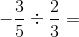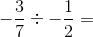# Dividing Negative Fractions

Instructions:

question 1 of 3

### Solve:Create Your Account To Take This Quiz

As a member, you'll also get unlimited access to over 79,000 lessons in math, English, science, history, and more. Plus, get practice tests, quizzes, and personalized coaching to help you succeed.

Try it risk-free for 30 days. Cancel anytime

### 1. Solve:### 2. What is the reciprocal of 13/15?

Create your account to access this entire worksheet
Quizzes, practice exams & worksheets
Certificate of Completion
Create an account to get started

This quiz/worksheet includes practice problems that can help you test your fluency when it comes to dividing negative fractions. You'll find both equation and word problems within.

## Quiz & Worksheet Goals

This quiz/worksheet consists of questions involving:

• Solving equations with negative fractions
• The reciprocal of 13/15
• Multiplying numerators and denominators
• Simplifying numbers

## Skills Practiced

• Problem solving - use acquired knowledge to solve practice equations involving negative fractions
• Information recall - access the knowledge you've gained regarding mathematic reciprocals
• Knowledge application - use your knowledge to divide negative fractions within a given word problem

• Distinguish the word 'negative' as it applies to mathematics
• Familiarize yourself with the rules of mixing positive and negative signs
• Review the steps for dividing negative fractions
• Explore provided examples of negative fraction division
Final Exam
Algebra for Kids
This course currently has no practice test
Chapter ExamWorking with Fractions for Elementary School
Status: Not Started

Support